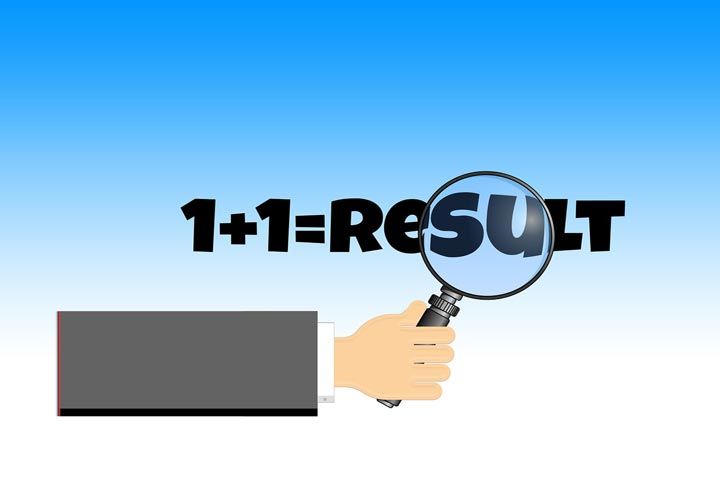# Your Child Will Be Able To Solve Mathematical Problems Related To Addition & Subtraction

Your kiddo, at this age of 6-7 years will be able to write and recognize numerals from 0 to 100. He is also able to count to 100 by ones, twos, fives, and tens. Your child is now ready to solve simple mathematical problems like addition and subtraction.

What you need to knowAt this point, your child will like to manipulate and play with objects. He likes it when a connection is made between his own environment and the math concepts that he is learning. Therefore, use real objects when teaching him how to add or subtract. For example, give your child three apples and then ask him to add one more apple. Ask him to count the total number of apples. The same method will work for subtraction too. You can use comparative language such as ‘greater than’, ‘less than’ to make your child understand the concepts of addition and subtraction better.Remember, your child needs to have a thorough understanding of numbers before he can perform arithmetic problems. For example, number 5, how many different ways can this number arrive through addition by pairing two numbers? Your child should be aware that  5 can arrive using number pairs of  5 and 0, 4 and 1, or  2 and 3.244

50

Shares